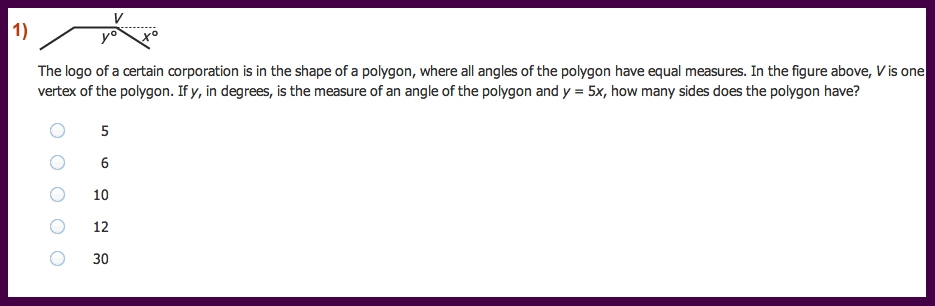# 5 Hardest GRE Questions

Posted by on March 25, 2015 in GRE | 22 comments

Since GRE test takers in Pakistan are always asking me for hard GRE questions, I have selected some of my favourite questions. All of these questions are 165+ level questions and similar questions have shown up on the GRE exam to high scorers. Answers to these questions will be available next week – meanwhile you can start solving these and you may put your answers in the comment box below. Good Luck!1.1. D
2.D
3.D

•That’s what I got

2.A1=12
A2=4214
A3=90

3.1)12
2)4214
3)70
4)E
5) tumult , relegate, vindictive
Please anyone shed some light on Verbal. Have my exam on 30th march :p

4.12
4214
70
E
Tumult relegate vindictive
Please shed some light on verbal. I have my exam on monday:p

5.1) x=180-y ===> x=180-5x
simplifying results x=30
sum of exterior angles always equal to 360 degree. Also number of exterior angles equal to number of sides. Hence, 360/30=12 sides, option D

2) Sum of numbers from 1-100=5050
Sum of even numbers b/w 25-63=836
Hence, 5050-836=4,214, Option D

3) 5C2 *4= 40 triangles, Option C

4) Just a fluke,.. may be option E
5) Idiosyncrasy, discern, vindictive

•3) (5C2*4C1)+(4C2*5C1)=70

•Yea. Abdullah, you’re only looking at an option where you take 2 points from the 5-point line and 1 point from the other line. But, you can also take 2 points out of the 4 points and the 3rd one from 5 points.

So, that’s what YASH does, he adds up the total count from both of the options.

6.1. 12
2. 4214
3. 70
4. C
5. Idiosyncrasy, discern, vindictive

7.The sum of interior angles of a regular polygon=(n-2)*180.
So the measure of each angle=(1/n)(n-2)*180.
In this problem, we have find n.
Since x+y=180
or x+5x=180
or 6x=180
or x=180/5=30
Therefore, y=5x=5*30=150.
Now (1/n)(n-2)*180=150
or (n-2)*180=150n
or 180n-360=150n
or 180n-150n=360
or 30n=360
Therefore n=360/30=12

•Can we assume x+y=180? that its a straight line?

•Yup

8.Answer to Q2: Even integers between 25 and 63 have to be omitted.
The number of integers between 25 and 63 =(1/2)(63-25)=(1/2)*38=19.
Sum of the omitted even omitted integers=(19/2)(2*26+(19-1)*2)=(19/2)2(26+18)=19*44
=836.
Sum of all numbers from 1 to 100=(100/2)(2*1+(100-1)1)=(50)(101)=5050.
Therefore, the sum of the integers from 1 and 100 inclusive, with the even integers between 25 and 63 omitted=5050-836=4214.

9.Answer to Q3: In order to form a triangle we have to take three points. We may either take any 2 points from 5-point line and the remaining 1 point from 4-p0int line, OR, we may take 1 point from the 5-point line and 2 points from the 4-point line.
Therefore, using combination formula we have 5C2*4C1+5C1*4C2=40+30=70.

10.for question 5: B, E, I

11.Question 4 is almost certainly D, correct? There is no reference to another industry besides the Machine-Tool industry and the passage states that the author “Examines the transformation of the Machine Tool industry” (history) and that he approaches the topic “From a Marxist perspective” (Ideological). I can’t find any evidence of a comparison or that this shows the origin of some industrial phenomenon, and choice C is a better description of the commentary that we are reading than it is “Forces of Nature” itself.

12.Are we to take from the answer choices in Question 3 that the GRE does not consider flat triangles to be triangles? 70 is only correct if we cannot take 3 points from the same line.

•Yes GRE does not consider flat or straight lines to be triangles

13.For question 5 BDH

14.What is the combination formula for #3? I understand the concept I just don’t have the formula.

15.what is the correct answers of question 5?

•Idiosyncrasy, relegate, vindictive